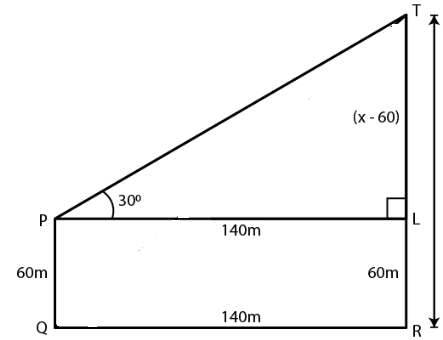Guru

# . The horizontal distance between two towers is 140 m. The angle of elevation of the top of the first tower when seen from the top of the second tower is 30. If the height of the second tower is 60 m, find the height of the first tower

• 0

sir this is the important  question from the book -ML aggarwal( avichal publication) class 10th , chapter20 , heights and distances
it is given that The horizontal distance between two towers is 140 m.
The angle of elevation of the top of the first tower when seen from the top of the second tower is 30.
If the height of the second tower is 60 m,
find the height of the first tower
question no 25 , heights and distances , ICSE board

Share

1. Consider the height of the first tower TR = x

It is given that

Height of the second tower PQ = 60 m

Distance between the two towers QR = 140 mConstruct PL parallel to QR

LR = PQ = 60 m

PL = QR = 140 m

So we get

TL = (x – 60) m

In right triangle TPL

tan θ = TL/PL

Substituting the values

tan 300 = (x – 60)/ 140

So we get

1/√3 = (x – 60)/ 140

By further calculation

x – 60 = 140/√3

Multiply and divide by √3

x – 60 = 140/√3 × √3/√3 = 140√3/3

We get

x = 140√3/3 + 60

x = (140 × 1.732)/ 3 + 60

x = 80.83 + 60

x = 140.83

Hence, the height of first tower is 140.83 m.

• 0